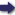(London :  Kegan Paul, Trench, Trübner & Co.,  1910.)

 Tools

## Search this bookPrev Page 45 Next``` CHAPTER LII. 45 and adhimdsct days, as the general lunar days are equal to the sum of universal solar days and universal ctdhi¬ mdsa days. Therefore the partial and the universal adhimdsct days stand in the same relation to each other as the two numbers written in two different places, there being no difference, whether they both mean months or days. The following rule of Ya'kub for the computation of Ya'kuVs: ° ^ • CI- • method for the partial unaratra days by means of the partial adhi- the compu- 1 • f 1 • -n 1 • PI- tationofthe masct months is found m all the manuscripts of his partial iima- . ratra days. book:— "The past adhimdsa, together with the fractions of the current adhimdsa, are multiplied by the universal iXna¬ rdtra days, and the product is divided by the universal solar months. The quotient is added to the adhimdsa. The sum is the number of the past imctrdtras." This rule does not, as I think, show that its author Criticism knew the subject thoroughly, nor that he had much confidence either in analogy or experiment. For the ctdhimdsa months which have passed of the cctturyitga up to our gauge-date are, according to the theory of Pulisa, 1,196,525^i-|-(^^. Multiplying this number by the itnardtrct of the caturyuga, we get the product 30,oii,6oo,o68,426y^-2V Dividing this number by the solar months, we get the quotient 578,927. Adding this to the ctdhimdsct, we get the sum 1,775,452. And this is not what we wanted to find. On the contrary, the number of iXnardtra days is 18,835,700. Nor is the product of the multiplication of this number by 30 that which we wanted to find. On the contrary, it is 53,263,560. Both numbers are far«»away from the truth. ```Prev Page 45 Next